# What are trinomials and Factoring Trinomials

If a polynomial has only three terms, then we call it a trinomial. In this course, we are going to discuss trinomials with an exponent of 2. In other words, we will only look at quadratic trinomials.

This tutorial covers factorization of trinomials with a leading coefficient of 1, trinomials with a common factor, and trinomials with a leading coefficient greater than one. This is a basic course in algebra that gives you a head start to further algebra at higher levels.

## How do we represent trinomials?

The general representation of trinomials is ax2+bx+c, where a is the leading coefficient. We will simplify examples with different values of a because that is usually where the trick is.

## How to factor a trinomial with a leading coefficient of one (a=1)?

Factorization of trinomials requires that you express them as a product of two-term polynomials (aka binomials). To successfully do that, let us first take a quick look at some of the mathematical identities that will make the simplification work easier.In many cases, we will be working in reverse, from right to left of the above expressions. If you can identify that any of these identities apply, then you can use them to simplify any trinomials. There is a trick in it, as we will see later in this tutorial. Enter your text here...

Example 1: Factorize x2+4x-32

Solution

By inspection, none of the three identities above can help us in factorizing the given expression. So, we are going to start working in reverse as follows:

We use the distributive property to help us in rewriting the middle term in a way that makes the expression easy to factor. The property states that when given a trinomial like the one we have in the example, you find two integers m and n such that their product equals c and sum equals b. Remember general representation of trinomials is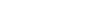In our case, a=1, b=4, and c=-32. So, we have a task of finding two integers whose product is 32, and the sum is 4. Tabulating your thoughts can help you find these two integers. Let’s do it!

Sum =  4

Product = -32

Remarks

1, 3

3

No

2, 2

4

No

5,  -1

-5

No

6, -2

-12

No

7, -3

-21

No

8, -4

-32

Yes

We finally got the pair at the bottom of the table. The two integers that we have been looking for are (8,-4). Now, let’s rewrite the expression using this information.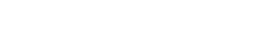The first group has a common factor, which is x. the last group also has 8 as the common factor. We can, therefore, factorize the expression.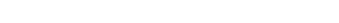Factoring (x-4) completes our process.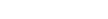Is there an easier way of finding the two numbers whose sum is equal to b and product equal to c? Unfortunately, the answer is no. However, there leads that can help you tabulate faster and get your pair within no time.

Tips:

1. 1
Inspect the value of c first. If it has a positive value, then the integer pair you are looking for are all positive. Otherwise, one integer of the pair is negative.
2. 2
Inspect the value of b. If both b and c are negative, then the integer pair has positive values. If b is negative and c is positive, then in integer pair has all negative values. If the value of b is positive and c is negative, then the integer pair with the greatest absolute value is positive. Lastly, if both c and b are negative, then the greatest absolute value is for the positive value.

Example 2: Factorize x2-10x+21

Solution

Since the value of c is +21, we know that all values of the pair are positive. By inspecting b, we see -10, meaning that all the integer values are negative. Using this knowledge, we can quickly develop a table. For this case, we can find the values by inspection since only the product of 3 and 7 gives 21. These values should all be negative for the sum to be equal to -10. Hence, the numbers are -7 and -3.
This is the factorization of the above expression: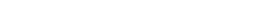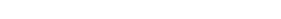## How to find a common factor?

If the value of the leading coefficient, a, is greater than one (a>1), then inspect the expression for a common factor. Pull it out and factor the other part the usual way.

Example 3: Factorize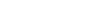Solution

By inspection, 3 is a common factor for all the terms. Let’s pull it out.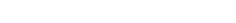Now, the term in the bracket is a simple trinomial that we can solve using the identity in equation (i).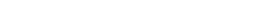The leading coefficient is greater than one, and there is no common factor

You will come across such situations where you have nothing to factorize and a>1. Do not panic, as there is a way of handling it. Let’s demonstrate that using an example.

Example 4: Factorize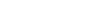Solution

Step 1: Find two integers whose sum equals b and product equals a×c.

In this case, a=3 and c =-2. The product, ac = 3 x -2 = -6. So, we will find two integers such that their sum is 1 and the product is -6 using the tips provided earlier.

The two integers that satisfy the two conditions are 3 and -2. Let’s rewrite the expression and factorize.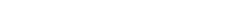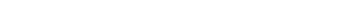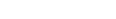## Remarks

The factorization of trinomials is a straightforward process. With practice, you can do it within the shortest time possible. As usual, you will need this knowledge in later chapters or your career. Remember that a trinomial is an expression in the form ax2+bx+c. Use the distributive property as learned in the tutorial to factorize any trinomials.

If this blog post was helpful, take a look at our online Math Tutoring courses which cover these topics and much more.  Our 1-on-1, live and online tutoring will help you master any math subject and ace your upcoming tests.